# Determine if the following series converges or diverges. Give reasons for your answer.1+2+3+ ... +nn=1

Question
4 views
check_circle

Step 1

Given series is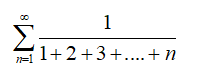We know that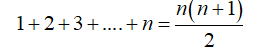Therefore, we have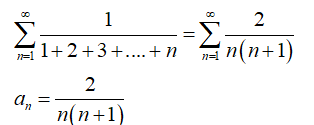Step 2

Now we use limit comparision test to check the convergence and divergence of given series, so we consider the following series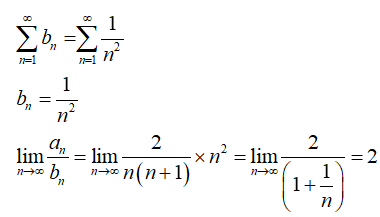Since the limit is non-zero finite, so both series converge and diverge together.

The series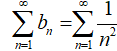converges by p-series test

Hence given series converges

...

### Want to see the full answer?

See Solution

#### Want to see this answer and more?

Solutions are written by subject experts who are available 24/7. Questions are typically answered within 1 hour.*

See Solution
*Response times may vary by subject and question.
Tagged in